# True Count Calculation — The Whole Story

Card counters know that before we bet or play using a balanced strategy, we must adjust the running count by the un-dealt cards. That is, convert the running count into a true count. Most explanations of true count calculation simply say that the running count is divided by the number of remaining decks in the shoe. Blackjack card counting books generally provide a simple example, like a running count of +6 divided by three remaining decks yields a true count of +2 — and they leave it at that. As a result many questions remain. The attempt here is to answer some of these questions.

### What is the true count divisor?

When using the Hi-Lo card counting strategy, we divide by the number of remaining decks. But this is not true of all strategies. For example:

• Revere Point Count and Hi-Lo Lite divide by remaining half-decks.
• The 1998 version of Zen divides by quarter-decks remaining. The original Zen divided by full-decks.
• The Mentor card counting strategy essentially divides by the number of double-decks remaining. More on this later.

Generally, there is not much difference in overall effectiveness. However, if you are not using fractional true counts for betting, there is a performance penalty for using half-decks in a level 1 strategy or quarter-decks in a level 2 strategy.

### How are non-integer results handled?

Suppose we have a running count of +3 and 2 remaining decks. 3/2=1.5. However, nearly all counters use integers for betting and indexes. There exist four methods of converting to integers:

• Truncate – For positive numbers, round down and for negative numbers round up. This is the method used in the 1994 and later editions of Professional Blackjack. 1.5 is rounded down to 1. -1.5 is rounded up to -1.
• Floor – Numbers are always rounded down. This is the method used in the early versions of Professional Blackjack. 1.5 is rounded down to 1. -1.5 is rounded down to -2. This is the most popular method used now.
• Round – After the True Count division, the result is rounded to the nearest integer. If the number is exactly between two integers, it is rounded up. 1.5 is rounded up to 2. -1.5 is rounded up to -1. This method is also common.
• Statistical Round – After the True Count division, the result is rounded to the nearest integer. If the number is exactly between two integers, it is rounded to the nearest even number. 1.5 is rounded up to 2. -1.5 is rounded down to -2.

Flooring and rounding are nearly equal in performance. Truncating is slightly inferior as it results in a very large percentage of true counts of zero, which in turn results in reduced precision. For best performance, you should use the method that was used when the indexes that you use were generated.

### How do we estimate remaining decks?

We need to divide by a number related to the number of decks remaining. But how do we obtain this number. Different players estimate the remaining cards in different manners as follows:

• Look at the shoe – This seems obvious as we are dividing by the number of remaining decks, and they are sitting in the shoe. However, this is not very accurate as all the cards are not visible and the cards are not always stacked tightly. Very few people use this method. On the other hand, Revere used to teach this method in his private classes precisely because so few people use this method and it doesn't require looking at the discard tray. Also, some tables now hide the cards in the discard tray.
• Subtract cards in discard tray – The most common method is to look at the discard tray and subtract from the starting number of decks. Of course this is not quite accurate for playing decisions since it ignores the cards on the table.
• Subtract cards dealt – In calculating the remaining cards, subtract the total number of cards dealt from the total cards including those on the table both seen and unseen. This is a bit more accurate, and more difficult. (Many Blackjack simulators incorrectly use this method.)
• Subtract cards seen – Only take into account the actual number of cards seen. That is the cards in the discard tray (minus unseen burn cards) plus only the cards on the table that you have seen. This is the most accurate and the most difficult. Few people use this method.
• Count hands – In single deck games, some people count the number of hands, multiply by 2.7 and subtract from 52.

By far, most people look at the discard tray.

### How closely must we estimate remaining decks?

That is, what is the resolution of the estimate. Very few people estimate remaining cards to the exact card. (Although some Blackjack simulators inaccurately assume this.) When playing shoes, most people estimate to the nearest remaining full-decks or half-decks remaining. When playing single deck, you should estimate by quarter-decks. In deeply-dealt games, you might want to be more accurate toward the end of the shoe. Alas we see few deeply-dealt Blackjack games these days. For best results, you should estimate the remaining decks to the same degree of accuracy that was used during generation of the indexes that you are using.

### Custom true count calculation

Some people use a table of divisors (or multipliers) instead of dividing by the remaining decks. For example, the Mentor count uses a table of multipliers used to convert the running count to a true count depending on a table of remaining decks that becomes more precise as the shoe is depleted. This is described in Blackjack Bluebook II by Fred Renzey.

### True count calculation by rounds

Yet another method is to look up a multiplier in a table based on the number of players and the round number. Then use that multiplier to convert the running count to a true count. This can be used in single deck play. This is described in Play Blackjack Like the Pros by Kevin Blackwood.

### How often do we calculate true counts?

Everyone recalculates before making a bet. Different players make additional recalculations differently as follows:

• Before Insurance – Recalculate before an Insurance bet. Counts all seen cards, including all of your hands in face down dealing.
• Before first decision – Recalculate before the first playing decision in each hand.
• Before every close decision – Recalculate for every playing decision that matters.

Recalcualtion for every decision is very important in single-deck Blackjack games. In shoe games, failing to recalculate results in a performance hit of 5%-10% depending on penetration.

### Is there any time that I do not calculate true count?

Some people use the running count for decisions with a zero index. For example, when you have a 16 vs. ten, the running count is actually a bit more accurate than the true count using some strategies.

And you thought it was simple.J

Share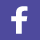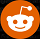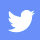News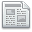Forum/Blog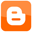Fav/EMailGambling problem, contact the National Council on Problem Gambling.